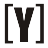How to Calculate Your Grade: Select tab Percentage / Letters or Points and insert the values in the box or select your Grade letter A+ – F in the Letters Tab (you can also add more row) and click the Calculate button.

Weighted grade = w1 × g1 + w2 × g2 + w3 × g3 + w4 x g4 + ….

Example:
1. Math class with grade of 75 and weight of 30%.
2. English class with grade of 80 and weight of 40%.
3. History class with grade of 82 and weight of 30%.

The weighted average grade is calculated by:

Weighted grade = 30% × 75 + 40% × 80 + 30% × 82 = 79.10 = C+YoosFuhl.com Would you like to receive notifications on latest updates? No Yes